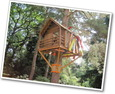# Unit Overview

Type of Unit: Concept; Project

Length of Unit: 18 days and 5 days for project

# Prior Knowledge

Students should be able to:

• Find the area of triangles and special quadrilaterals.
• Use nets composed of triangles and rectangles in order to find the surface area of solids.
• Find the volume of right rectangular prisms.
• Solve proportions.

# Lesson Flow

• After an initial exploratory lesson that gets students thinking in general about geometry and its application in real-world contexts, the unit is divided into two concept development sections: the first focuses on two-dimensional (2-D) figures and measures, and the second looks at three-dimensional (3-D) figures and measures.
• The first set of conceptual lessons looks at 2-D figures and area and length calculations. Students explore finding the area of polygons by deconstructing them into known figures. This exploration will lead to looking at regular polygons and deriving a general formula. The general formula for polygons leads to the formula for the area of a circle. Students will also investigate the ratio of circumference to diameter ( pi ). All of this will be applied toward looking at scale and the way that length and area are affected. All the lessons noted above will feature examples of real-world contexts.
• The second set of conceptual development lessons focuses on 3-D figures and surface area and volume calculations. Students will revisit nets to arrive at a general formula for finding the surface area of any right prism. Students will extend their knowledge of area of polygons to surface area calculations as well as a general formula for the volume of any right prism. Students will explore the 3-D surface that results from a plane slicing through a rectangular prism or pyramid. Students will also explore 3-D figures composed of cubes, finding the surface area and volume by looking at 3-D views.
• The unit ends with a unit examination and project presentations.
Subject:
Mathematics, Geometry
Level:
Middle School
7
Tags:
• 3d
• Geometry
• Models
• Polygons
• Surface Area

Lesson 2

· Scissors (one pair for each student)

· Rulers (one for each student)

· Print copies of Area of Regular Polygons (one for each student). Print out, cut apart, and laminate if desired.

Lesson 3

• Graphing chart paper that is 12-inch by 12-inch with 1-inch spacing (one for each student)
• A 12-inch by 12-inch piece of cardboard (one for each student)
• Glue (one for each pair of students)
• Ruler (one for each student)

Lesson 4

· String (several spools for the class)

· Rulers (one for each student)

· Scissors (one for each student)

Lesson 6

Ruler (one for each student)

Lesson 7

· Centimeter grid paper (one for each student)

· Ruler (one for each student)

· Compass (one for each student)

Lesson 8

· Base sheets for project (one for each student)

· Square foot cardboard pieces to mount base sheets on (one for each student)

· Rulers (one for each pair of students)

· Protractors (one for each pair of students)

Lesson 16

Students’ centimeter grid layout to draw the nets

Lesson 17

· Origami cubes

· Cardboard

Lesson 18

· Centimeter grid layout

· 1-inch grid paper

· Rulers

· Scissors, tape, protractors (available as needed)

Unit 4 Zooming In On Figures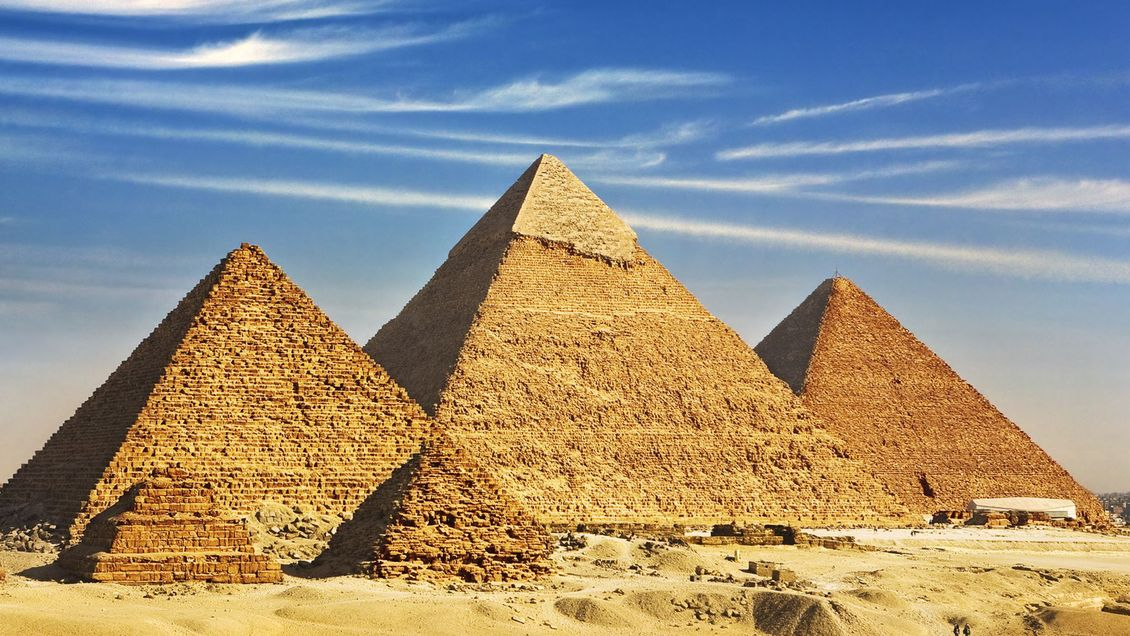•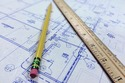Lesson 1

House Plans
•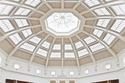Lesson 2

Polygons
•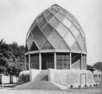•••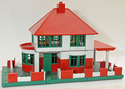Lesson 6

Scale
•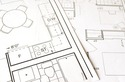•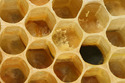•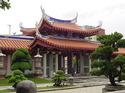••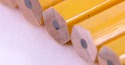•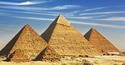•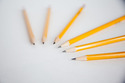•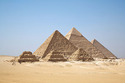•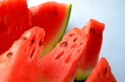•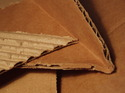Lesson 16

Unit Review
•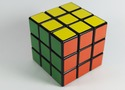••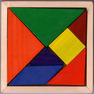•